# Compound Interest Formula

## Definition

Compound interest is the cumulation of interest to the principal sum of a loan or deposit

## Formula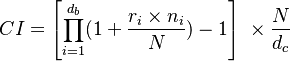$CI = \left[ \prod_{i=1}^{d_b} (1 + \frac{r_i \times n_i}{N}) - 1 \right] \ \times \frac{N}{d_c}$

Where

•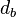$d_b$ is the number of business days in the interest period
•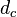$d_c$ is the number of calendar days in the interest period
•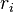$r_i$ is the interest rate applicable on business day i
•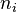$n_i$ is the number of calendar days for which rate ri applies (on most days, ni will be 1, but on a Friday it will generally be 3, and it will also be larger than 1 on the business day before a holiday).
• N is the market convention for quoting the number of days in the year.

]